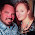## Monday, February 24, 2014

### 33 Watch: Pizza Numerology!

The Pizza is credited with originating from Naples, Italy.
• Naples = 5+1+7+3+5+1 = 22
• Italy = 9+2+1+3+7 = 21 = 3
• Naples, Italy = 22 3
Notice the number 223 is the number 322 reversed; a number associated with Yale's elite Skull and Bones secret society.  Skull and Bones is fixated on numerology, and refers to their members as 'Bonesmen'.  I've often wondered where this number 322 might have originated from; perhaps Naples, Italy is a clue.  If you consider the fact that the oldest University in the world originates from Italy, and the other elder Universities such as Oxford and Cambridge incorporate numerology into their names, the thought doesn't seem too far off of the mark.
• Bonesmen = 2+6+5+5+1+4+5+5 = 33
That number 33 is associated with religions of the world, and has direct ties back to ancient Rome, which we will visit in a moment.  Christianity, which spread under the Roman Empire, had its central figure, Jesus Christ, put to death at the age of 33; only after he performed 33 miracles.  With all that in mind, let us examine the numerology of  'Pizza', which also originates from Rome and Italy.
• Pizza = 7+9+8+8+1 = 33
Could it be a coincidence?  Possibly... but the number 33 has deeps ties in Italy, as mentioned before.  Two of the oldest basilicas from ancient Rome, that can still be visited and appreciated today, are Santa Prassede and Santa Cecilia.  Let us quickly examine the numerology of these words.
• Santa = 1+1+5+2+1 = 10 = 1
• Prassede = 7+9+1+1+1+5+4+5 = 33
• Cecilia = 3+5+3+9+3+9+1 = 33
At the site of Santa Prassede is the famous San Zeno Chapel.
• San Zeno = 1+1+5+8+5+5+6 = 31
• ...at Santa Prassede = Prassede = 7+9+1+1+1+5+4+5 = 33
• The ranging degrees of Freemasonry are 31-33
Prassede was one of three female Saints from her era.
• Agnes = 1+7+5+5+1 = 19 = 10 = 1
• Pudenziana = 7+3+4+5+5+8+9+1+5+1 = 48 = 12 = 3
• Prassede = 7+9+1+1+1+5+4+5 = 33
• Prassede and Pudenziana were sisters.
• 19+48+33 = 100

1.2.